Mechanics

# Kepler laws of planetary motion definition and equation

Kepler laws of planetary motion are expressed as:(1) All the planets move around the Sun in the elliptical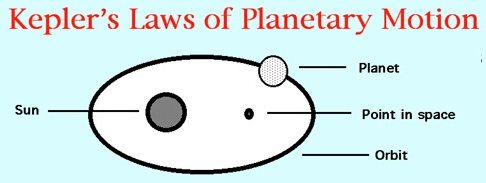orbits, having the Sun as one of the foci. (2) A radius vector joining any planet to  Sun sweeps out equal areas in equal intervals of time. (3) The square of the period of any planet about the sun is proportional to the cube of the planet’s mean distance from the sun.

## Three laws of planetary motion

Using newton’s laws of motion and law of universal gravitation, we can understand and analyze the behavior of all the bodies in the solar system: the orbits of the planets and comets about the sun and of natural and artificial satellites about their planets. We make two assumptions that simplify the analysis:

1. We consider the gravitational force only between the orbiting body and the central body (the sun), ignoring the perturbing effect of the gravitational force of other bodies (such as other planets).
2. We assume that the central body is so much more massive than the orbiting body that we can ignore its motion under their mutual interaction. In reality, both objects orbit around their common center of mass, but if one object is very much more massive than the other, the center of mass is approximately at the center of the more massive body. Exceptions to this second assumption will be noted.

The empirical basis for understanding the motions of the planets is Kepler’s three laws, and we now show how these laws are related to the analytical results of newton’s laws.

## Kepler’s first law of planetary motion

“All planets move in elliptical orbits, with the sun at one focus.”

Explanation: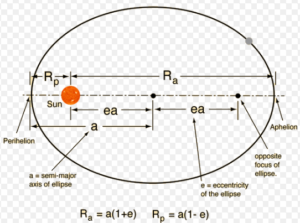Consider a planet of mass ‘m’ moving in such an orbit around the sun, whose mass is M.We assume that M>>m so that the center of mass of the planet sun system is approximately at the center of mass of the planet sun system is approximately at the center of the sun. The orbit is described by two parameters: the semi-major axis ‘a’ and the eccentricity. The distance from the center of the ellipse to either focus is ‘ea’.The maximum distance Ra of the planet from the sun is called aphelion, similarly, the closest distance RP is called perihelion.

From the figure we see that:

Ra=a +ea =a(1+e) ———-(1)

Rp=a-ea=a(1-e) ————–(2)

A circular orbit is a special case of an elliptical orbit with e=0.
For a circular orbit, from equation (1) and (2) we get,

Ra=Rp=a

## Kepler’s second law of planetary motion

“A line joining any planet to the sun sweeps out equal areas in equal times.”

Or

“Areal velocity of the planet around the sun is constant.”

## Kepler’s 2nd law equation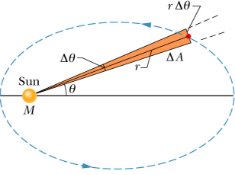Consider a planet of mass is moving in an elliptical orbit around the sun. The sun and the planet are separated by distance r. Consider the small area ∆A covered in a time interval ∆t, as shown in the figure. The area of the wedge is approximately the area of a triangle with base ‘rΔθ‘ and height ‘r’.Since the area of a triangle is one-half of the base times the height.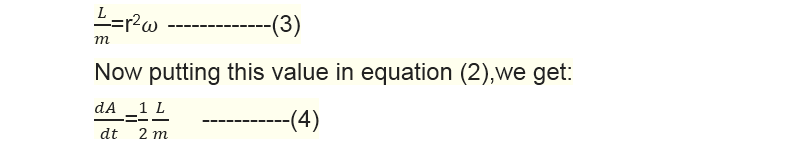Where dA/dt is called areal velocity. Since angular momentum ‘L’ and mass of the planet are constant.

## Kepler’s third law of planetary motion

“The square of the period of any planet about the sun is proportional to the cube of the planet’s mean distance from the sun.”

## Kepler’s 3rd law equation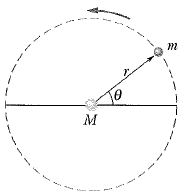Let us prove this result for circular orbits. Consider a planet of mass ‘m’ is moving around the sun of mass ‘M’ in a circular orbit of radius ‘r’ as shown in the figure. The gravitational force provides the necessary centripetal force to the planet for circular motion. Hence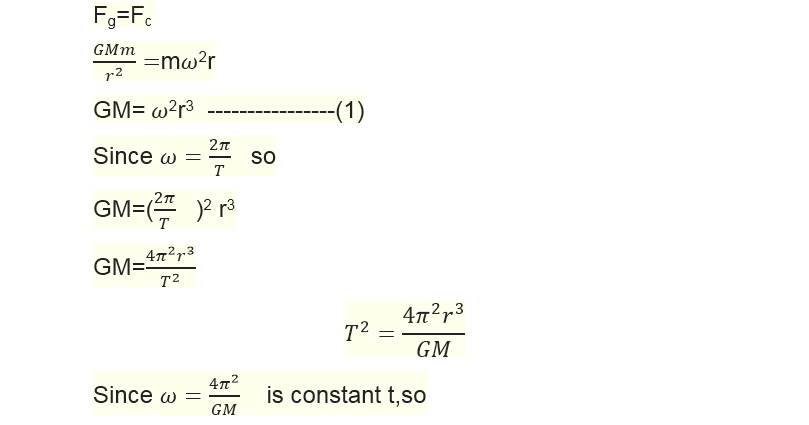T2    r3

A similar result is obtained for elliptical orbits with radius ‘r’ replaced by semi-major axis ‘a’ given by the relation:

T2    a3